Question

Consider a unity-feedback control system with the following open-loop transfer G(s)

Consider a unity-feedback control system with the following open-loop transfer G(s) = 1/(s3+0.2s2 +s+1)

Draw a Nyquist plot of G(s). You may draw the simple Nyquist plot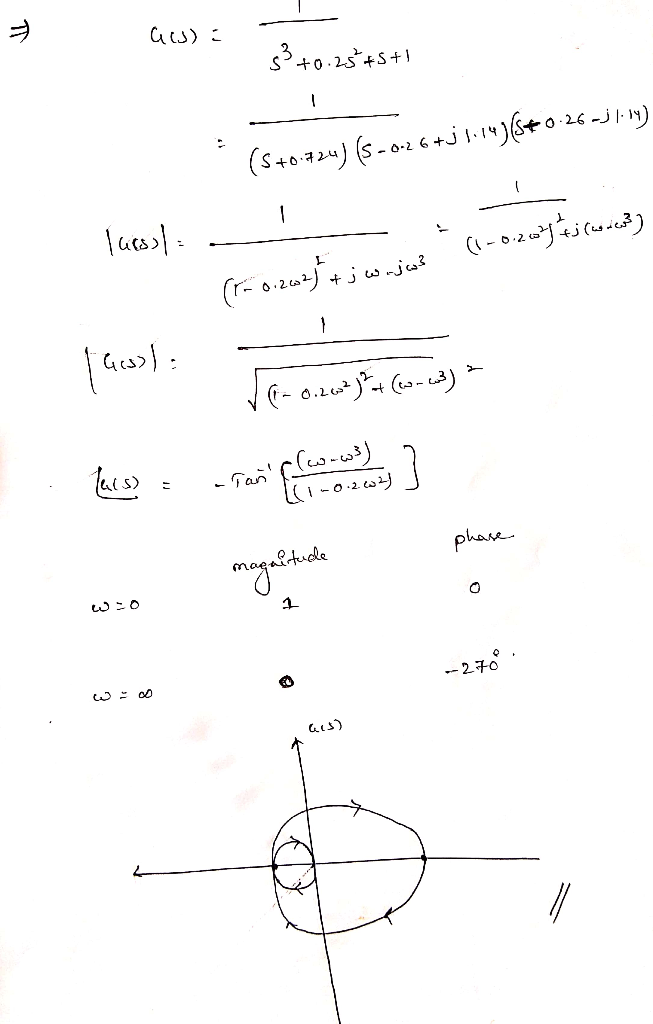Earn Coins

Coins can be redeemed for fabulous gifts.

Similar Homework Help Questions
• 4. Consider a unity-feedback control system with the following open-loop transfer function: G(s)3 Sketch a Nyquist...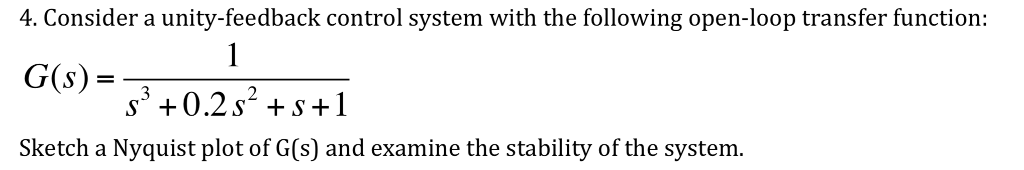4. Consider a unity-feedback control system with the following open-loop transfer function: G(s)3 Sketch a Nyquist plot of G(s) and examine the stability of the system.

• A unity feedback control system has the following open loop transfer function, K L(s) .If K 10, evaluate the stability...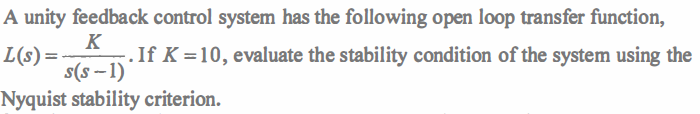A unity feedback control system has the following open loop transfer function, K L(s) .If K 10, evaluate the stability condition of the system using the s(s-1) Nyquist stability criterion. A unity feedback control system has the following open loop transfer function, K L(s) .If K 10, evaluate the stability condition of the system using the s(s-1) Nyquist stability criterion.

• 2. A unity feedback system has the following open-loop transfer function -0.5s + 0.5 G(s)i a)...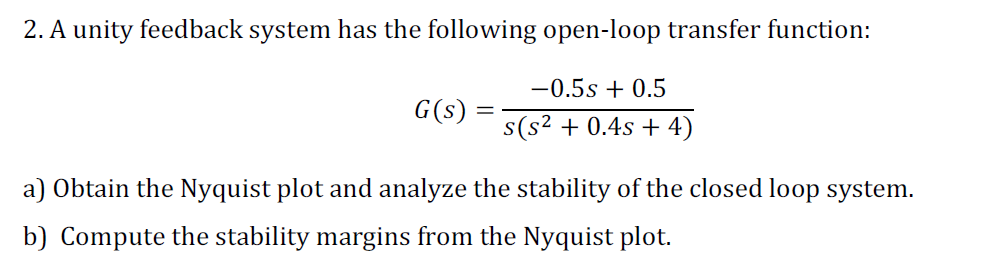2. A unity feedback system has the following open-loop transfer function -0.5s + 0.5 G(s)i a) Obtain the Nyquist plot and analyze the stability of the closed loop system b) Compute the stability margins from the Nyquist plot.

• 7. Consider a unity feedback control system with open-loop transfer function G(s) = k 5 s...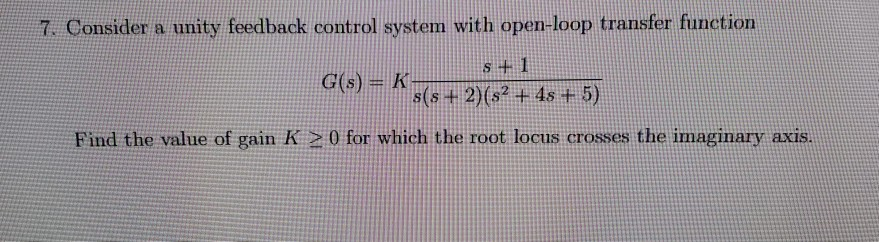7. Consider a unity feedback control system with open-loop transfer function G(s) = k 5 s + 2)(52 + 4s + 5) Find the value of gain K > 0 for which the root locus crosses the imaginary axis.

• Consider a unity feedback control system with open loop transfer function KG(G) s(s+2)(s + 6) 1....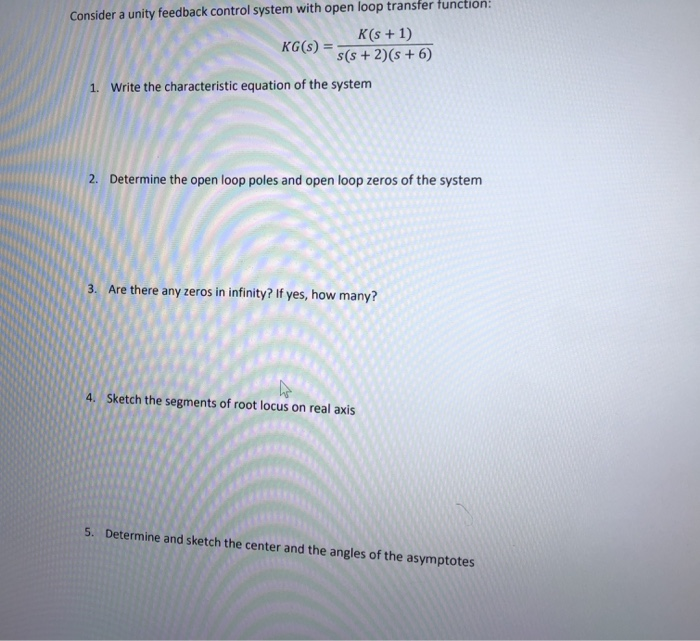Consider a unity feedback control system with open loop transfer function KG(G) s(s+2)(s + 6) 1. Write the characteristic equation of the system 2. Determine the open loop poles and open loop zeros of the system 3. Are there any zeros in infinity? If yes, how many? 4. Sketch the segments of root locus on real axis 5. Determine and sketch the center and the angles of the asymptotes

• 2. Consider a unity feedback control system whose open-loop transfer function is K(s-2) G(s) (s+1)(s +6s...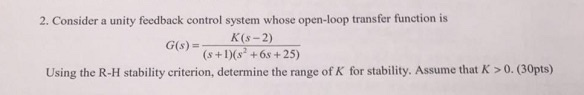2. Consider a unity feedback control system whose open-loop transfer function is K(s-2) G(s) (s+1)(s +6s +25) Using the R-H stability criterion, determine the range of K for stability. Assume that K > 0. (30pts)

• PD & PID controller design Consider a unity feedback system with open loop transfer function, G(s)...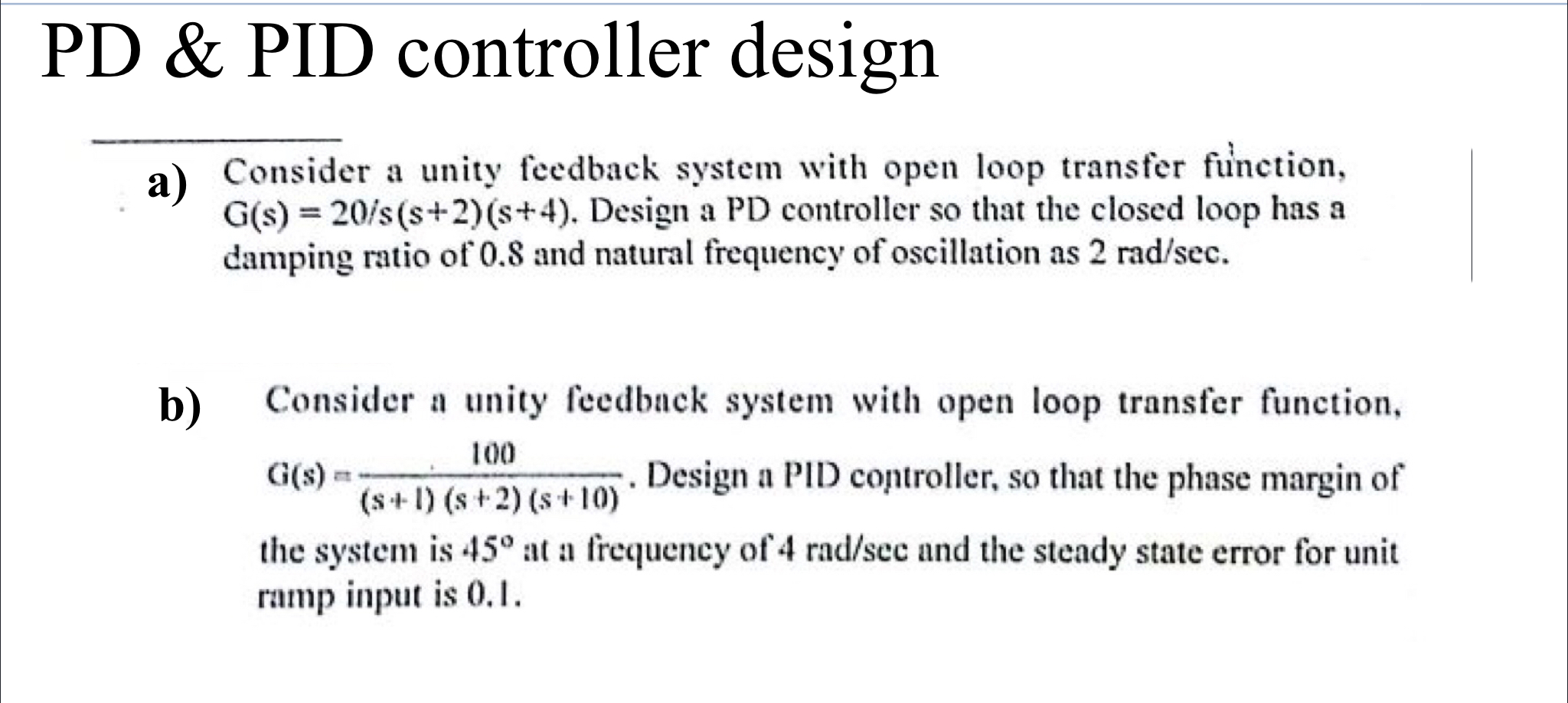PD & PID controller design Consider a unity feedback system with open loop transfer function, G(s) = 20/s(s+2)(8+4). Design a PD controller so that the closed loop has a damping ratio of 0.8 and natural frequency of oscillation as 2 rad/sec. b) 100 Consider a unity feedback system with open loop transfer function, aus. Design a PID controller, so that the phase margin of (S-1) (s + 2) (s+10) the system is 45° at a frequency of 4 rad/scc and...

• Due Date: April 20, 2 Problem 2: Consider a unity-feedback control system with the following open-loop...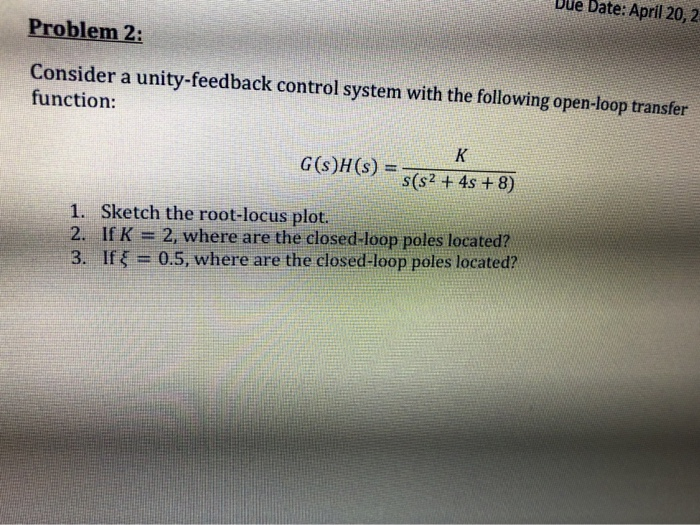Due Date: April 20, 2 Problem 2: Consider a unity-feedback control system with the following open-loop transfer function: K G(s)H(s) = s(s2 + 4s + 8) 1. Sketch the root-locus plot. 2. IfK 2, where are the closed-loop poles located? 3. If x = 0.5, where are the closed-loop poles located?

• A unity gain negative feedback system has an open-loop transfer function given by 4. s) =...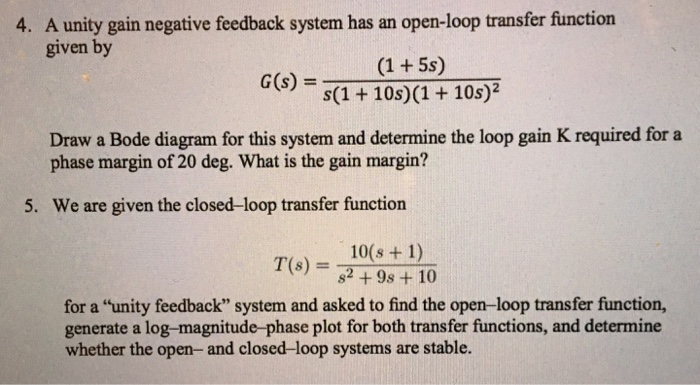A unity gain negative feedback system has an open-loop transfer function given by 4. s) = s(1 + 10s)(1 + 10s)? Draw a Bode diagram for this system and determine the loop gain K required for a phase margin of 20 deg. What is the gain margin? 5. We are given the closed-loop transfer function 10(s + 1) T(s) = 82+98+10 for a "unity feedback" system and asked to find the open-loop transfer function, generate a log-magnitude-phase plot for both...

• QUESTION 4 A unity feedback system has open-loop transfer function G(S). Polar plot of G(jw) is...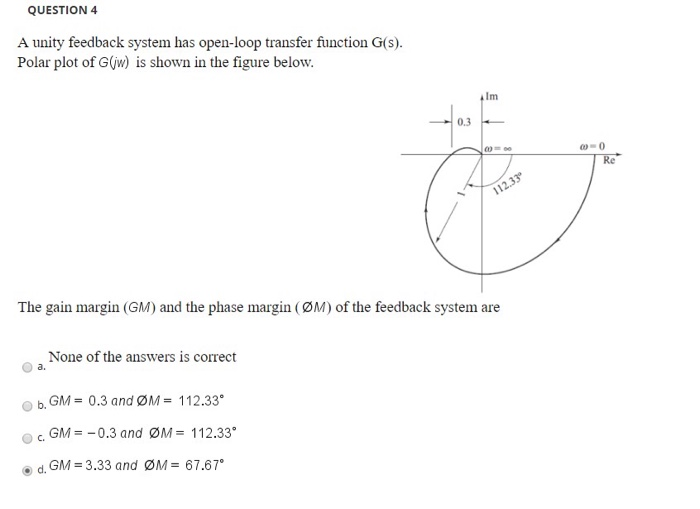QUESTION 4 A unity feedback system has open-loop transfer function G(S). Polar plot of G(jw) is shown in the figure below. The gain margin (GM) and the phase margin (ØM) of the feedback system are None of the answers is correct b. GM = 0.3 and ØM = 112.33º O GM = -0.3 and ØM = 112.33 d. GM = 3.33 and ØM = 67.67° QUESTION 8 A unity feedback system as open-loop transfer function G(s) = 5(1 +ST) The...